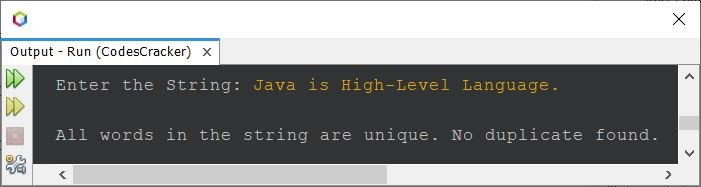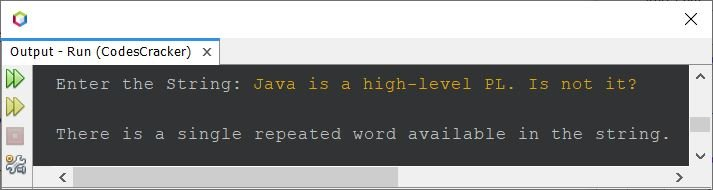# Java Program to Count Number of Repeated Words in a String

For example, if the given/entered string is java is robust java is portable, then the output will be 2. Because two words, i.e., "java" and "is", repeated in the given string.

The question is, write a Java program to count the number of repeated words in a string. The string must be received by user at run-time of the program. Here is its answer:

```import java.util.Scanner;

public class CodesCracker
{
public static void main(String[] args)
{
String mystr, word;
int len, i, count, j, k, repeated=0;
Scanner s = new Scanner(System.in);

System.out.print("Enter the String: ");
mystr = s.nextLine();

String wordArray[] = mystr.split(" ");
len = wordArray.length;

for(i=0; i<len; i++)
{
word = wordArray[i];
count = 1;
for(j=(i+1); j<(len-1); j++)
{
if(word.equals(wordArray[j]))
{
count++;
for(k=j; k<(len-1); k++)
{
wordArray[k] = wordArray[k+1];
}
len--;
j--;
}
}
if(count>1)
repeated++;
count = 0;
}

System.out.println("\nTotal Repeated Words = " +repeated);
}
}```

Here is its sample run with user input java is robust java is portable as string to find and print total number of repeated words in it:The problem with above program is, if user enters a string in which the same word with its character(s) in uppercase and lowercase will get treated as different words. Also the above program is not allowed for multiple whites spaces. Therefore I've created another program after modifying the above one. That is,

```import java.util.Scanner;

public class CodesCracker
{
public static void main(String[] args)
{
int repeated=0;
Scanner s = new Scanner(System.in);

System.out.print("Enter the String: ");
String mystr = s.nextLine();

mystr = mystr.toLowerCase();
String wordArray[] = mystr.split("\\s+");
int len = wordArray.length;

for(int i=0; i<len; i++)
{
String word = wordArray[i];
int count = 1;
for(int j=(i+1); j<(len-1); j++)
{
if(word.equals(wordArray[j]))
{
count++;
for(int k=j; k<(len-1); k++)
{
wordArray[k] = wordArray[k+1];
}
len--;
j--;
}
}
if(count>1)
repeated++;
count = 0;
}

if(repeated==0)
System.out.println("\nAll words in the string are unique. No duplicate found.");
else if(repeated==1)
System.out.println("\nThere is a single repeated word available in the string.");
else
System.out.println("\nThere are " +repeated+ " repeated words found in the string.");
}
}```

Here is its sample run with user input Java is High-Level Language.Here is another sample run with user input, Java is a high-level PL. Is not it?That duplicate word is "is", because it occurs more than one time. The first one is "is", and the second one is "Is".

Java Online Test

« Previous Program Next Program »